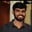Related Tags

omega
bigoh
theta
communitycreator

# Asymptotic notations and their propertiesBuchiredddypalli Koushik

Asymptotic notations are used to analyze and determine the running time of an algorithm.

There are three main types of asymptotic notations:

• Big-oh notation: Big-oh is used for upper bound values.
• Big-Omega notation: Big-Omega is used for lower bound values.
• Theta notation: Theta is used for average bound values.

## Properties of Asymptotic Notation

### General properties

• If f(n) is Big-Oh(g(n)), then a*f(n) is Big-Oh(g(n))

• If f(n) is Omega(g(n)), then a*f(n) is Omega(g(n))

### Reflexive

• If f(n) is given, then f(n) is Big-Oh(f(n))

### Transitive

• If f(n) is Big-Oh(g(n)) and g(n) is Big-Oh(h(n)), then f(n)=Big-oh(h(n))

### Example

$f(n)=n g(n)=n^2 and h(n)=n^3$

then $n = Big-oh(n^2)$ $n^2 = Big-oh(n^3) -> n = Big-oh(n^3)$

### Symmetric

If f(n) is theta(g(n)), then g(n) is theta(f(n))

Suppose $f(n)=n^2; g(n)=n^2$

$f(n)=theta(n^2)$

$g(n)=theta(n^2)$

### Transpose symmetric

If f(n) = Big-Oh(g(n)), then g(n) is Omega(f(n))

### Example

$f(n)=n$ and $g(n)=n^2$

Tmhen n is $big-oh(n^2)$ and $n^2$ is $omega(n)$

RELATED TAGS

omega
bigoh
theta
communitycreator

CONTRIBUTORBuchiredddypalli Koushik
RELATED COURSES

View all Courses

Keep Exploring

Learn in-demand tech skills in half the time